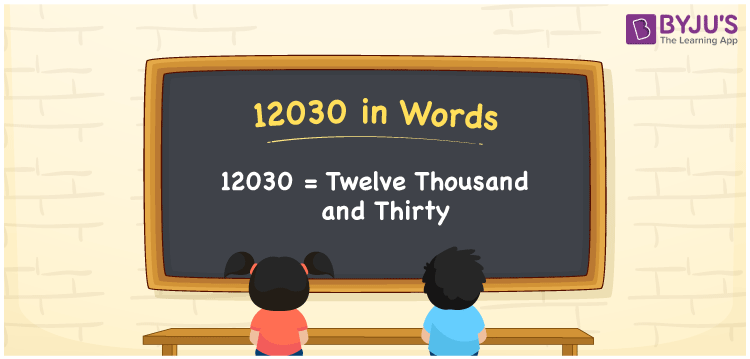# 12030 in Words

12030 in words is twelve thousand and thirty. The number 12030 is a natural number and it exists between 12029 and 12031. It is also a cardinal number. For example, the cost of chairs purchased is Rs. 12030. Now, let us understand the procedure of writing the number 12030 in words with the help of the place value system in detail.

 12030 in Words: Twelve Thousand and Thirty. Twelve Thousand and Thirty in Numerical Form: 12030.

## 12030 in English Words## How to Write 12030 in Words?

The place values of 12030 up to five digits are given below:

 Ten-thousands Thousands Hundreds Tens Ones 1 2 0 3 0

The expanded form of 12030 is as follows:

= 1 × Ten thousand + 2 × Thousand + 0 × Hundred + 3 × Ten + 0 × One

= 1 × 10000 + 2 × 1000 + 0 × 100 + 3 × 10 + 0 × 1

= 10000 + 2000 + 30

= 12030

= Twelve thousand and thirty

Hence, 12030 in words is twelve thousand and thirty.

12030 in words – Twelve thousand and thirty

Is 12030 an odd number? – No

Is 12030 an even number? – Yes

Is 12030 a perfect square number? – No

Is 12030 a perfect cube number? – No

Is 12030 a prime number? – No

Is 12030 a composite number? – Yes

## Frequently Asked Questions on 12030 in Words

Q1

### Write 12030 in words.

12030 in words is twelve thousand and thirty.

Q2

### Simplify 12000 + 30, and express it in words.

Simplifying 12000 + 30, we get 12030. Hence, 12030 in words is twelve thousand and thirty.

Q3

### Is 12030 a prime number?

No, 12030 is not a prime number